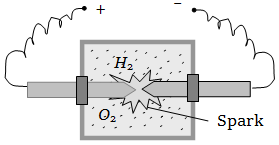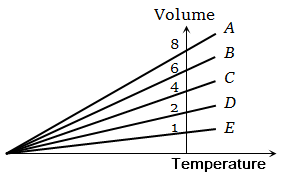Inside a cylinder having insulating walls and closed at ends is a movable piston, which divides the cylinder into two compartments. On one side of the piston is a mass m of a gas and on the other side a mass 2 m of the same gas. What fraction of volume of the cylinder will be occupied by the larger mass of the gas when the piston is in equilibrium ? Consider that the movable piston is conducting so that the temperature is the same throughout

1.  $\frac{1}{4}$

2.  $\frac{1}{3}$

3.  $\frac{1}{2}$

4.  $\frac{2}{3}$

High Yielding Test Series + Question Bank - NEET 2020

Difficulty Level:

The diameter of oxygen molecules is  The Vander Waal's gas constant 'b' in ${\mathrm{m}}^{3}/\mathrm{mol}$ will be

1.  $3.2$

2.  $16$

3.  $32×{10}^{-4}$

4.  $32×{10}^{-6}$

High Yielding Test Series + Question Bank - NEET 2020

Difficulty Level:

The temperature of the mixture of one mole of helium and one mole of hydrogen is increased from $0°\mathrm{C}$ to $100°\mathrm{C}$ at constant pressure. The amount of heat delivered will be

1.  600 cal

2.  1200 cal

3.  1800 cal

4.  3600 cal

High Yielding Test Series + Question Bank - NEET 2020

Difficulty Level:

A vessel contains a mixture of one mole of oxygen and two moles of nitrogen at 300 K. The ratio of the average rotational kinetic energy per ${\mathrm{O}}_{2}$ molecule to that per ${\mathrm{N}}_{2}$ molecule is

1.  1 : 1

2.  1 : 2

3.  2 : 1

4.  Depends on the moments of inertia of the two molecules

High Yielding Test Series + Question Bank - NEET 2020

Difficulty Level:

A vessel contains 14 gm (7 moles) of hydrogen and 96 gm (3 moles) of oxygen at STP. A chemical reaction is induced by passing electric spark in the vessel till one of the gases is consumed. The temperature is brought back to it's starting value 273 K. The pressure in the vessel is1.  0.1 atm

2.  0.2 atm

3.  0.3 atm

4.  0.4 atm

High Yielding Test Series + Question Bank - NEET 2020

Difficulty Level:

A gas mixture consists of 2 mole of oxygen and 4 mole of argon at temperature T. Neglecting all vibrational modes, the total internal energy of the system is

1.  4 RT

2.  15 RT

3.  9 RT

4.  11 RT

High Yielding Test Series + Question Bank - NEET 2020

Difficulty Level:

A jar contains a gas and few drops of water at T K. The pressure in the jar is 830 mm of mercury. The temperature of jar is reduced by 1%. The saturated vapour pressure of water at the two temperatures are 30 mm and 25 mm of mercury. Then the new pressure in the jar will be

1.  917 mm of Hg

2.  717 mm of Hg

3.  817 mm of Hg

4.  None of these

Concept Questions :-

Ideal gas
High Yielding Test Series + Question Bank - NEET 2020

Difficulty Level:

Molar specific heat of oxygen at constant pressure  At constant volume, 5 mol of oxygen is heated from  the quantity of heat required is approximately

1.

2.

3.

4.

High Yielding Test Series + Question Bank - NEET 2020

Difficulty Level:

One mole of an ideal gas requires 207 J heat to raise the temperature by 10 K when heated at constant pressure. If the same gas is heated at constant volume to raise the temperature by the same 10 K, the heat required is

1.  198.7 J

2.  29 J

3.  215.3 J

4.  124 J

(Given the gas constant )

High Yielding Test Series + Question Bank - NEET 2020

Difficulty Level:

The expansion of an ideal gas of mass m at a constant pressure P is given by the straight line D. Then the expansion of the same ideal gas of mass 2m at a pressure P/ 2 is given by the straight line , where number on graphs indicate slope , is-1.  E

2.  C

3.  B

4.  A